# All About Try Catch Block in C++

## All About Try-Catch Block in C++ with Examples

In this article, I am going to discuss Try-Catch Block in C++ with Examples. Please read our previous article where we discussed How to Throw and Catch Exception Between Functions in C++ with Examples.

##### Try Catch Block in C++

Now let us discuss the try and catch blocks in C++. Suppose we have written some statements, let’s say 4 lines of code inside the try block, and when there is an exception in any line, from that line, it will come to the catch block. Suppose there is an exception of type integer, so the catch block with the integer argument will catch the integer exception. And inside the catch block, we can give a proper massage. For a better understanding, please have a look at the below image.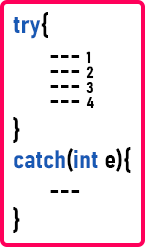For a better understanding, please have a look at the below code.

```#include <iostream>
#include<conio.h>
using namespace std;
int main ()
{
int num = 1;

try
{
if (num == 1)
// throwing integer value as exception
throw 1;
else
cout << "Value" << num << endl;
}
catch (int ex) // to catch Integer Exceptions
{
cout << "Integer Exception" << endl;
}

return 0;
}
```

Output: Integer Exception

Suppose the try block is now throwing two different types of exceptions. For example, if the num value is equal to 1, then throw integer exception and if the num value is equal to 2, then throw float exception. Then the integer catch block will not handle both the exceptions. It will handle only integer exceptions. For a better understanding, please have a look at the below example.

```#include <iostream>
#include<conio.h>
using namespace std;
int main ()
{
int num = 2;

try
{
if (num == 1)
// throwing integer value as exception
throw 1;
else if (num == 2)
// throwing float value as exception
throw 10.5f;
else
cout << "Value" << num << endl;
}
catch (int ex)	// to catch Integer Exceptions
{
cout << "Integer Exception" << endl;
}

return 0;
}
```

Output: terminate called after throwing an instance of ‘float’

How to resolve the above problem. The answer is multiple catch blocks?

##### Multiple Catch Blocks in C++:

So, we can have one catch block to handle the integer exception and another catch block to handle the float exception. So, we can have more than one catch block for a try block in C++ as shown in the below image.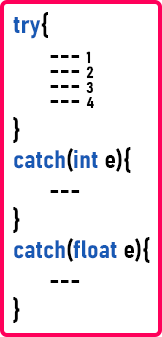So, if there is an exception of the integer type, it will go to the first catch block and if there is an exception of the float type, then it will go to the second catch block. For a better understanding, please have a look at the below example code.

```#include <iostream>
#include<conio.h>
using namespace std;
int main ()
{
int num = 2;

try
{
if (num == 1)
// throwing integer value as exception
throw 1;
else if (num == 2)
// throwing float value as exception
throw 10.5f;
else
cout << "Value" << num << endl;
}
catch (int ex)	// to catch Integer Exceptions
{
cout << "Integer Exception" << endl;
}
catch (float ex) // to catch Floating Exceptions
{
cout << "Float Exception" << endl;
}

return 0;
}
```

Output: Float Exception

Note: The point that you need to remember is that we can have multiple catch blocks for a single try block in C++ language to handle different types of exceptions.

##### Generic Catch Block (Catch-All) in C++:

Suppose within the try block at line number 4, there is some exception and we don’t know which type of exception it is. Then how to handle this? To handle the unknown exceptions, we need to use a generic catch block i.e. one catch block with (…) as shown in the below image.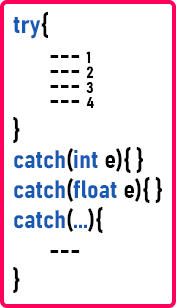This type of catch block in C++ is called catch-all. So, when we mentioned (…), this is called an ellipse. And if you are using ellipse, then it can handle any type of exceptions thrown in the try block. For a better understanding, please have a look at the below example.

```#include <iostream>
#include<conio.h>
using namespace std;
int main ()
{
int num = 3;

try
{
if (num == 1)
// throwing integer value as exception
throw 1;
else if (num == 2)
// throwing float value as exception
throw 10.5f;
else if (num == 3)
throw "Unknown Exception";
else
cout << "Value" << num << endl;
}
catch (int ex)	// to catch Integer Exceptions
{
cout << "Integer Exception" << endl;
}
catch (float ex)	// to catch Float Exceptions
{
cout << "Float Exception" << endl;
}
catch (...)	// to catch all Exceptions
{
cout << "Unknown Exception" << endl;
}

return 0;
}
```

Output: Unknown Exception

So, we have learned two important things: first, we can have multiple catch blocks for each type of data; and second, we can have a catch block for all types of exceptions.

##### Throwing User-Defined Type in C++:

Suppose there is no float type exception in the try block, the exception is of type myExp. So, can we write myExp in the catch block? Yes. We can write my exception in the try block as shown in the below image.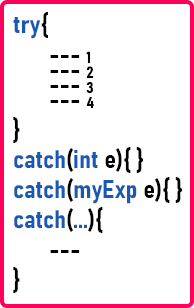For a better understanding, please have a look at the below example code. In the below example, we are throwing the class MyExp from the try block and handling that exception in the catch block.

```#include <iostream>
#include<conio.h>
using namespace std;
class myExp
{
};
int main ()
{
int num = 2;
try
{
if (num == 1)
// throwing integer value as exception
throw 1;
else if (num == 2)
// throwing myExp exception
throw myExp();
else if (num == 3)
throw "Unknown Exception";
else
cout << "Value" << num << endl;
}
catch (int ex)	// to catch Integer Exceptions
{
cout << "Integer Exception" << endl;
}
catch (myExp e)	// to catch myExp Exceptions
{
cout << "myExp Exception" << endl;
}
catch (...)	// to catch all Exceptions
{
cout << "Unknown Exception" << endl;
}

return 0;
}
```

Output: myExp Exception

Now, suppose the exception is in the last line of the try block and the exception is of type float, or character, or any other type. Then this will be handled by this catch-all (…) block. So, in the above diagram, we have defined only two catch blocks (one for integer and another for myExp) and, for the remaining exceptions, there is a common catch block that will handle the exceptions.

##### Multiple Catch Blocks vs Catch-All Block:

So, always writing multiple catch blocks is better instead of using catch-all. If you are using catch-all, it means you are not interested in giving a clear message to the user. But according to industry standards, for every type of error, a separate message related to the error must be present.

##### Can we write catch-all first and then other catch blocks?

No, we cannot write the catch blocks in the following manner.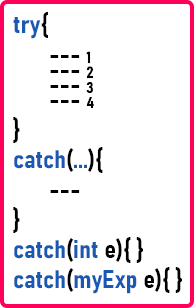If we write catch-all first, then all the exceptions will be handled here only. The lower catch blocks will never be executed. So, the catch-all block must be the last block. For a better understanding, please have a look at the below example.

```#include <iostream>
#include<conio.h>
using namespace std;
class myExp
{
};
int main ()
{
int num = 2;
try
{
if (num == 1)
// throwing integer value as exception
throw 1;
else if (num == 2)
// throwing myExp exception
throw myExp();
else if (num == 3)
throw "Unknown Exception";
else
cout << "Value" << num << endl;
}
catch (...)	// to catch all Exceptions
{
cout << "Unknown Exception" << endl;
}
catch (int ex)	// to catch Integer Exceptions
{
cout << "Integer Exception" << endl;
}
catch (myExp e)	// to catch myExp Exceptions
{
cout << "myExp Exception" << endl;
}

return 0;
}
```
###### Output: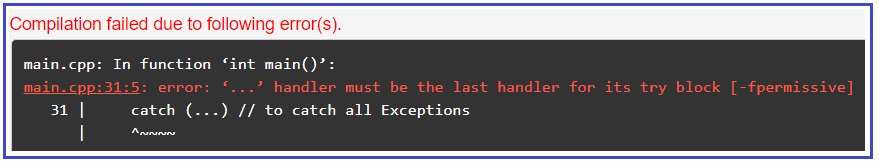When you try to run the above code, you will get an error and this is because we have the catch-all block before other catch blocks. So, that’s it. We have learned about multiple catches.

##### Can we have a Try Block inside a Try block?

Yes, we can have a try block inside another try block in C++. The following diagram shows the syntax of writing nested try blocks in C++.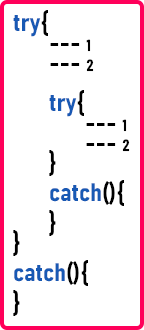So, this is a try and catch block inside another try block. So, we can do nesting of try and catch blocks in C++. So that’s it about nested try and catch.

##### Example to Understand Try-Catch Blocks in C++:

Now, suppose we have two classes as follows:
class MyExp1{};
class MyExp2 : public MyExp1{};

So, we have these two classes. And MyExp2 is publically inherited from the MyExp1 class. So, MyExp1 is a parent class and MyExp2 is a child class. Please have a look at the following image. Here, we have a try block and there is a possibility that both the types of exceptions (MyExp1 and MyExp2) are thrown from the try block. Then we have written two catch blocks to catch both the exceptions.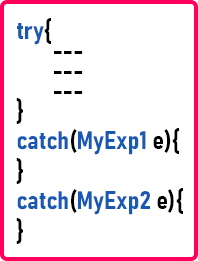As you can see, we have written catch blocks for both types of exceptions. So, is this the correct format of catch block? No. We have written the catch block for the parent class first and then for the child class. We must write catch blocks for the child class first and then for the parent class as shown in the below image.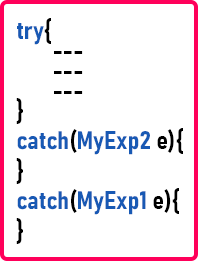So, we have to take care of this. For a better understanding, please have a look at the below example.

```#include <iostream>
using namespace std;

class myExp1
{
};

class myExp2:public myExp1
{
};

int main()
{
try
{
throw myExp1();
}
catch (myExp2 e)
{
cout << "myExp2 Catch Block" << endl;
}
catch (myExp1 e)
{
cout << "myExp1 Catch Block" << endl;
}
catch (...)
{
cout << "All Catch Block" << endl;
}
return 0;
}
```

Output: myExp1 Catch Block